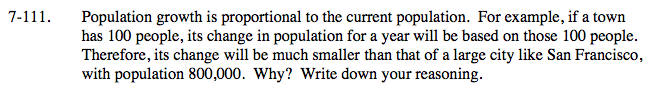### Home > CALC > Chapter 7 > Lesson 7.3.1 > Problem7-111

7-111.
1. Population growth is proportional to the current population. For example, if a town has 100 people, its change in population for a year will be based on those 100 people. Therefore, its change will be much smaller than that of a large city like San Francisco, with population 800,000. Why? Write down your reasoning. Homework Help ✎

2.$\text{Let }P(t) =\text{ current population and }\frac{dP}{dt}=\text{ population growth}.$

If population growth is proportional to current population, set up a proportional equation to model this statement.
Remember the constant of proportionality.

What 'type' of function must it be?
Is it: linear, quadratic, trigonometric, exponential, or something else?